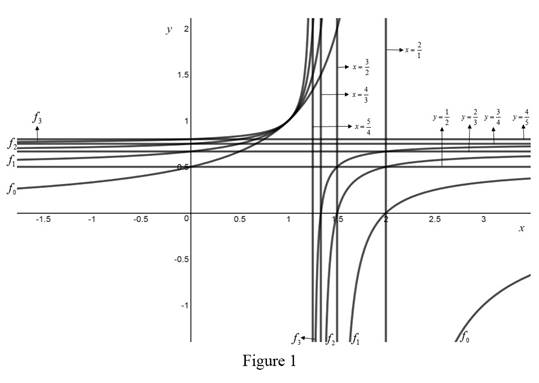# The expression for f n ( x ) .### Single Variable Calculus: Concepts...

4th Edition
James Stewart
Publisher: Cengage Learning
ISBN: 9781337687805### Single Variable Calculus: Concepts...

4th Edition
James Stewart
Publisher: Cengage Learning
ISBN: 9781337687805

#### Solutions

Chapter 1, Problem 20P

(a)

To determine

## To find: The expression for f n ( x ) .

Expert Solution

Solution:

The expression is, f n ( x ) = n + 1 n x n + 2 ( n + 1 ) x .

### Explanation of Solution

Given:

f 0 ( x ) = 1 ( 2 x ) .

f n + 1 f 0 ( f n ( x ) )  for n = 0 , 1 , 2...

Calculation:

Base case: n = 1 .

To prove that the statement is true for n = 1 .

f n + 1 = f 0 ( f ( x ) 1 ) f 2 = f 0 ( f ( x ) 1 )

Therefore, the statement is true for n = 1 .

Induction hypothesis: n = k

Assuming that the claim is true for n = k .

That is, f k + 1 ( x ) = f 0 ( f k ( x ) )

Inductive step: n = k + 1

To prove the statement is true for n = k + 1 .

f 0 ( x ) = 1 ( 2 x )

Substitute n = 1 ,

f 1 ( x ) = f 0 ( 1 ( 2 x ) )

f 1 ( x ) = 1 2 ( 1 ( 2 x ) ) f 1 ( x ) = 2 x 3 2 x

Substitute n = 2 ,

f 2 ( x ) = 1 2 ( 2 x 3 2 x ) f 2 ( x ) = 3 2 x 6 4 x ( 2 x ) f 2 ( x ) = 3 2 x 4 3 x

Substitute n = 3 ,

f 3 ( x ) = 1 2 ( 3 2 x 4 3 x ) f 3 ( x ) = 4 3 x 8 6 x ( 3 2 x ) f 3 ( x ) = 4 3 x 5 4 x

Thus, the general formula is f n ( x ) = n + 1 n x n + 2 ( n + 1 ) x

The claim is true for n = k .

f k ( x ) = k + 1 k x k + 2 ( k + 1 ) x

To prove the statement is true for n = k + 1 .

f k + 1 ( x ) = f 0 ( f k ( x ) ) f k + 1 ( x ) = f 0 ( k + 1 k x k + 2 ( k + 1 ) x ) f k + 1 ( x ) = 1 2 k + 1 k x k + 2 ( k + 1 ) x f k + 1 ( x ) = k + 2 ( k + 1 ) x k + 4 2 ( ( k + 1 ) x ) k + 1 k x

f k + 1 ( x ) = k + 2 ( k + 1 ) x k + 3 ( k + 2 ) x

Therefore, that is true for k + 1 .

Thus, f n ( x )   is true for all positive integer n .

(b)

To determine

Expert Solution

### Explanation of Solution

Graph:

The graph of the functions are shown below in Figure 1.From Figure 1, if the value of n increased, then the vertical asymptotes moves to y = 1 .

If the value of n   increased, then the horizontal asymptotes moves to x = 1 .

The x - intercept for f n + 1   is the value of vertical asymptote f n

The y - intercept for f n   is the value of vertical asymptote f n + 1 .

### Have a homework question?

Subscribe to bartleby learn! Ask subject matter experts 30 homework questions each month. Plus, you’ll have access to millions of step-by-step textbook answers!